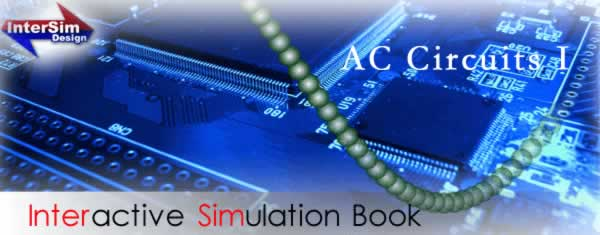Product Lineup

##### Chapter 1

You learn,

symbols you used in electric circuits,

various quantities which appear in electric circuits,

various formulas for electric engineering, and

how to use Oscilloscope.

### Chapter 2

You learn,

the relationship among a period, frequency and

angular frequency,

the relationship between maximum value and

effective value,

how to calculate a magnitude and a phase with

oscilloscope,

how to express an AC voltage or current as an

instantaneous value or in vector, and

the relationship between AC voltage and current of

a resistor, coil and capacitor.

### Chapter 3

You learn,

how to convert a complex number between rectangular

and polar forms.

how to convert an AC voltage or current from

instantaneous value to complex number,

the concept of impedance and admittance,

how to calculate the voltage and current of a circuit in a

complex form, and

how to calculate the complex voltage, complex current,